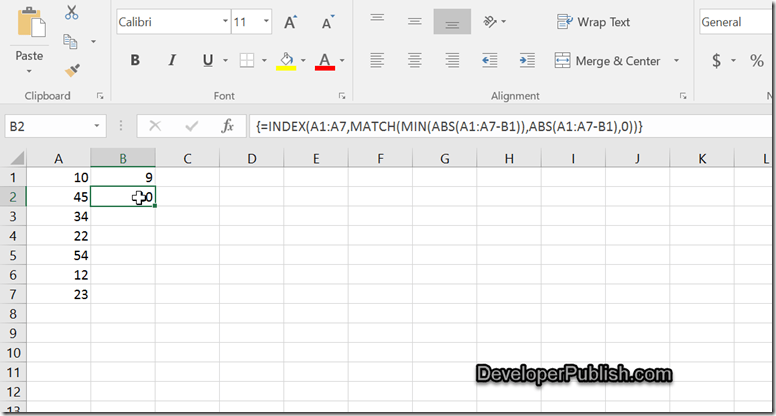# Finding the nearest value in Microsoft Excel

Assume that you have a range of numbers in a column and you are required to find out the nearest value or the closest value to the specified number from the range.

# How to find the nearest value in Microsoft Excel ?

You can use the array formula combined with the functions MATCH and MIN to find the same.

Assume that the cells A1:A1 has the values and you have the specified number in the cell “B1” Ex: 9 to find the nearest number and display it in cell B2.

Enter the following formula and press the keyboard shortcut key Ctrl + Shift + Enter.

=INDEX(A1:A7,MATCH(MIN(ABS(A1:A7-B1)),ABS(A1:A7-B1),0))